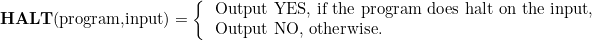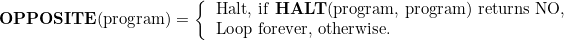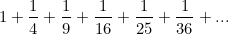## MATHS BITE: Sierpinski Number

A Sierpinski number is an odd natural number k such thatis not prime for all natural numbers n. In 1960, Sierpinski proved that there are infinitely many odd integers k with this property, but failed to give an example. Numbers in such a set with odd k and k < 2^n are called Proth numbers.

78557, 271129, 271577, 322523, 327739, 482719, 575041, 603713, 903983, 934909,….

-List of some known Sierpinski Numbers

### Sierpinski Problem

The Sierpinski problem asks what the smallest Sierpinski number is. In 1967, Sierpinski and Selfridge conjectured that 78557 is the smallest Sierpinski number. To show this is true, we need to show that all the odd numbers smaller than 78557 are not Sierpinski numbers, i.e. for every odd k below 78557 there is a positive interger n such thatis prime. There are only five numbers which have not been eliminated:

k = 21181, 22699, 24737, 55459, and 67607

### Numberphile Video

Find out more here. M x

## Waring’s Problem

### Statement:

Take any whole number k that is greater than or equal to 2.  Show that there is some number s (that is allowed to depend on k) so that every positive whole number is a sum of s kth powers.

### Proof

This problem was first solved by David Hilbert, and was then later proved by G.H. Hardy and John Littlewood in a different way. In this case, the second proof actually gave a lot more insight to the problem. To solve Waring’s problem as it has been stated you don’t need to give an s, you only need to show that one exists.  Finding the smallest s that works is a much more challenging (although arguably more interesting) problem. Using the, now named, Hardy-Littlewood circle method, Hardy and Littlewood wrote down an expression that approximated the number of ways to write N as a sum of s kth powers:The proof of Waring’s problem comes from that fact that since this must be positive and also be a whole number, there must be some way to write N as a sum of s kth powers.

M x

(Sorry for missing a post last week, my university work is getting a bit hectic so posts may be more sporadic!)

## How to Solve It

In 1945, Hungarian mathematician George Pólya wrote an extremely successful book called How to Solve It, which sold over one million copies and has been translated into 17 languages. In this book, Pólya identifies the four basic principles of problem solving.

### 1. Understand the Problem

Firstly, you must understand the problem you are tackling.  Although this may seem like common sense, it is often overlooked; the amount of times I’ve been staring at a problem for hours because I haven’t fully understood what it is asking! Consider asking the following questions:

• What is the unknown? What are the data? What is the condition?
• What are you asked to find or show?
• Can you restate the problem in your own words?
• Do you understand all the words used in stating the problem?

For some areas in maths, such as mechanics, drawing a labelled figure can often be extremely helpful to visualise what is going on.

### 2. Devise a Plan

There are many different suitable ways to solve a problem, as mentioned by Pólya. However, it is important to choose an appropriate strategy, which is a skill learnt by solving many problems. Some strategies include:

• Guess and check
• Use symmetry
• Consider special cases
• Solve an equation or use a formula
• Look for a pattern
• Solve a simpler problem
• Work backwards
• Eliminate possibilities

When choosing a strategy, it may help to consider whether you have solved a related problem already or whether you can think of a familiar problem that has the same or similar unknown.

### 3. Carry out the Plan

Once you have devised the plan, this step is simple if you already have the necessary skills, often only required care and patience -try to avoid silly mistakes!

Persist with the plan that you have chosen, only discarding it after multiple failed attempts. Then, return to step 2.

### 4. Look back

Pólya highlights how a lot can be gained from looking back and reflecting on the work you have done, asking yourself what worked and what didn’t. This way you can implement strategies that were successful for future problems that are similar.

M x

## VIDEO: Napkin Ring Problem

If you were to core a sphere (remove a cylinder from it), you are left with a shape that looks like a napkin ring. This is a “bizarre” shape, as if you have two napkin rings with the same height, they will have the same volume regardless of the size of the initial spheres that they came from. How do you prove this?Here’s a few hints to try and solve it yourself before watching the Vsauce video below which reveals the answer:

• There are a few variables that need to be found: the height of the napkin ring, the radius of the starting sphere and the radius of the cylinder. Using these variables you can find a volume equation.
• You don’t need to find the volume of the whole napkin ring in one go. This is because, as the two napkin rings have to be the same height, it’s enough to show that any slice of the napkin rings has to have the same area. If every pair of slices has the same area, then the napkin rings have the same volume.

M x

## Kakeya Needle Problem

The Kakeya needle problem asks whether there is a minimum area of a region in the plane in which a line segment of width 1 can be freely rotated through 360°, where translation of the segment is allowed.

This question was first posed for convex regions in 1917 by mathematician Sōichi Kakeya. It was shown by Gyula Pál that the minimum area for convex regions is achieved by an equilateral triangle of height 1 and area 1/√3.

Kakeya suggested that the minimum area, without the convexity restriction, would be a three-pointed deltoid shape. However, this is false.

### Besicovitch Sets

Besicovitch was able to show that there is no lower bound >0 for the area of a region in which a needle of unit length can be turned around. The proof of this relies on the construction of what is now known as a Besicovitch set, which is a set of measure zero in the plane which contains a unit line segment in every direction.

One can construct a set in which a unit line segment can be rotated continuously through 180 degrees from a Besicovitch set consisting of Perron trees.

However, although there are Kakeya needle sets of arbitrarily small positive measure and Besicovich sets of measure 0, there are no Kakeya needle sets of measure 0.

M x

## The Josephus Problem

The Josephus Problem is a problem in computer science based on a counting-out game. The problem is named after Flavius Josephus, a Jewish-Roman historian from the 1st century, who tells the story like this:

“A company of 40 soldiers, along with Josephus himself, were trapped in a cave by Roman soldiers during the Siege of Yodfat in 67 A.D. The Jewish soldiers chose to die rather than surrender, so they devised a system to kill off each other until only one person remained. (That last person would be the only one required to die by their own hand.)

All 41 people stood in a circle. The first soldier killed the man to his left, the next surviving soldier killed the man to his left, and so on. Josephus was among the last two men standing, “whether we must say it happened so by chance, or whether by the providence of God,” and he convinced the other survivor to surrender rather than die.”

This story gives rise to an interesting maths problem: If you’re in a similar situation to Josephus, how do you know where to stand so you will be the last man standing?

M x

## The Halting Problem

To understand the process of computation, regardless of the technology available, we need to reduce it to its most basic elements. One way to model computation is to consider an input, operated on by a program which produces some output.

In 1936, Alan Turing constructed such a model and considered the question of determining whether a program will finish running or will continue to run forever i.e. halt. This is known as the Halting Problem.

Let us suppose that we write a program, let’s call it HALT, that, given another program and its associated input, can determine whether running that program with the input will halt or not. This can be represented in the following way:Note that we do not have to consider how this program works, it is merely sufficient to assume that there does exist such a program. Now, consider a new program, call it OPPOSITE which does the opposite of halt. In other words, it analyses programs running with themselves as the input, acting in the following way:Now, what happens when we run OPPOSITE on itself?

If OPPOSITE(opposite) halts, then it must loop forever, but if it loops forever then it must halt. Hence, there is a contradiction, highlighting how the program HALT cannot exist.

This was an incredible result. Firstly, in this proof a computer and a program were mathematically defined, paving the way to the creation of the Turing Machines. Furthermore, Turing had found a program whose existence is logically impossible, and showed that knowing whether a program halts on some input is undecidable.

“It is one of the first examples of a decision problem.”

I have included a video by Computerphile which I think describes the problem and proof very well:

M x

## The Basel Problem

The Basel Problem is a problem in number theory posed by Pietro Mengoli in 1644, and solved by Leonhard Euler in 1734. As the problem remained open for 90 years, Euler’s solution brought him immediate fame at the age of 28.

The Basel Problem asks the result of the sumi.e. the sum of the inverse of the square numbers.As there are infinitely many square numbers, this sum has infinitely many terms. It is possible to find the ‘result’ of this sum due to the fact that the sum converges to a particular limiting value as the denominator approaches infinity. Part of the difficulty of solving this series is because it converges very slowly; when n = 106, the result is only accurate to 5 decimal places.

Euler found that the result had the value of:But how did he do this?

Firstly, recall the Taylor series expansion of sin(x):If we divide by x, this gives us:Applying the Weierstrass factorisation theorem, which states that “entire functions can be represented by a product involving their zeroes” we obtain the expression:If we multiply out this product and collect all x2 terms we get:In the expansion of sin(x)/x the coefficient of x2 is -1/(3!). By comparing coefficients and noting that they must be equal on each side, we can equate this as:Hence, reaching a final result ofSources: 1 | 2 | 3

M x

## 7 Days of Christmas: Day 7!

One of the most controversial problems in mathematics this year that went viral was a problem for Singaporean secondary school students asking, “When is Cheryl’s Birthday?”

To figure this out, we’re given the following information:So how do we solve it?

We must remember two facts:

• Albert is told that it’s either in May, June, July or August.
• Bernard is told that it’s either on the 14, 15, 16, 17, 18 or 19

Albert: I don’t know when Cheryl’s birthday is, but I know that Bernard doesn’t know too.

We can deduce that the only way Bernard could know the date with a single number, would be if Cheryl had told him 18 or 19, since of the ten date options only these numbers appear once (as May 19 and June 18). For Albert to know that Bernard does not know, Albert must therefore have been told July or August, since this rules out Bernard being told 18 or 19.

Bernard: At first I don’t know when Cheryl’s birthday is, but now I know.

Bernard has deduced that Albert has either August or July and if he knows the full date, he must have been told 15, 16 and 17 as they refer to one specific month, but 14 could be either month.

Albert: Then I also know when Cheryl’s birthday is.

Therefore, Albert has figured out that the possible dates are July 16, August 15 and August 17. For him to now know, he must have been told July, since if he had been told August he would not know which date for certain is Cheryl’s birthday.

Hence, the answer is July 16.